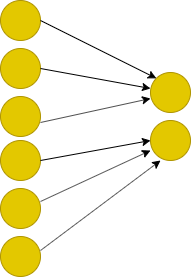# Linear layer with local connections

I want to implement linear regression in Pytorch with sparse connections. To build such a network I cannot use nn.Linear because it is a densely connected layer. I have first tried to make a binary matrix with 0’s and 1’s to indicate the presence and absence of connections. But it won’t work in my case because it is not computationally efficient. I am looking for a sparse weight matrix or sparse linear layer type solutions. I would be really grateful if you would help me in this regard. I have attached a screenshot also to explain what im trying to do.2 Likes

How is binary mask matrix not efficient? That should only be the case with 1)no gpu 2)huge weight matrix (megabytes) 3)big sparsity, like 90%. If your scenario is similar to that, you can try torch.sparse.mm.

Im working with images. Consider 128x128 pixels color image, A linear transformation for these size images can be represented by a matrix of size 49152 x 49152.

So, in this case dense layer would not work for me. Thats why im looking look for sparse solution. Can you please explain how can i use torch.sparse.mm instead of nn.Linear?

are you looking for matrix operations over matrices that are sparse by block ? That would surely reduce you dimensionality problem.

In that case, what about using several `Linear` layers that are not sparse instead of a large sparse`Linear` layer?

Can’t you express the needed connectivity with convolutions?

Anyway, about sparse, I just checked if it works:

``````i = torch.LongTensor([[0, 1, 1], [2, 0, 2]])
v = nn.Parameter(torch.FloatTensor([3, 4, 5]))
w = torch.sparse.FloatTensor(i,v, torch.Size([2,3]))
w.to_dense()
tensor(cpu,(2, 3)[[0., 0., 3.],